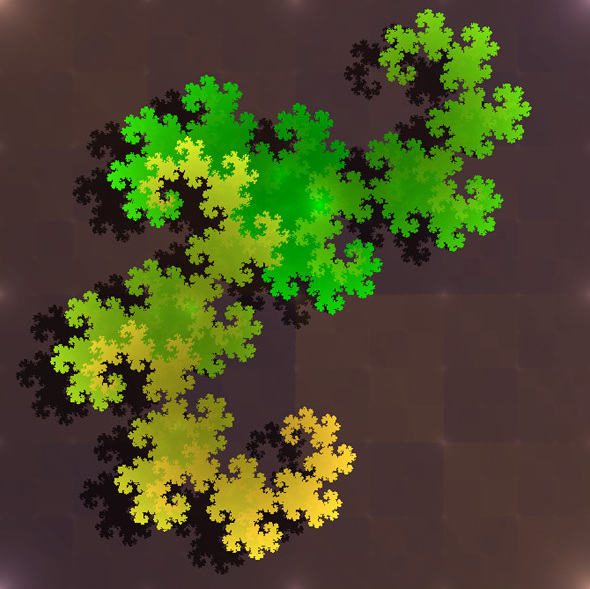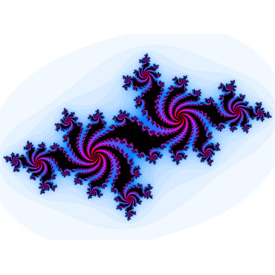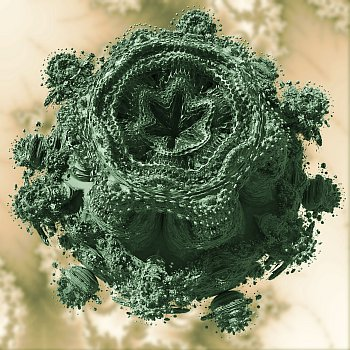## Fundamentals of Fractals 2: What is a fractal?There are many answers to this question, but let’s take a look at the common definitions:
”A set of points whose fractal dimension exceeds its topological dimension” -Paul Bourke
”A fractal is a rough or fragmented geometric shape that can be split into parts, each of which is a reduced-size copy of the whole” – Wikipedia
”A geometric pattern that is repeated at ever smaller scales to produce irregular shapes and surfaces that cannot be represented by classical geometry” – Dictionaries

A fractal usually have these features:

• Self-similar
• Finite structures
• Simple definition
• Recursive

This means that a fractal is a self-similar geometry, the geometry is built by parts that is similar with itself at a finite scale, based on a simple and recursive definition. This will make the fractal look very similar at an infinite magnification, meaning that you can zoom into infinity and loose yourself in the beauty of complex numbers.

Examples of fractals
The following table contain images of various and common fractals:

 Sierpinski gasket(Image from Wikipedia)Sierpinski carpet(Image from Wikipedia)Menger sponge(Image from Wikipedia)Heighway dragon(Image from Wikipedia)Koch snowflake(Image from Wikipedia)Julia Set(image from Paul Nylander)Mandelbrot3d Julia Set(Image from Paul Nylander)3D Mandelbrot set(Image by Krzysztof Marczak)Mandelbox(Image from Wikipedia)This is only a fraction of the various forms of fractals that exist, and in later tutorials, we will dive into a few, and learn how to generate them using HSLS and DirectX11. If your not using DX11, don’t worry, the algorithm and shaders will be easy to convert into other technologies as well.

How to generate fractals
We typically got four different techniques for generating fractals.

 Iterated function systems Created with a fixed geometric replacement rule. Typically, the Sierpinski carpet and gasket, Kock Snowflake, Heighway dragon and Menger sponge is created this way. Escape – time fractals Fractals that are defined by using recurrence or a formula at each point. Typically, the Mandelbrot and Julia set is created this way. Random fractals Created by a stochastic process. Strange attractors Created by the iteration of maps.

This tutorial will be updated over time. Feel free to give me some feedback on mistakes or if I’m missing something important.

This entry was posted in DirectX, DirectX11, Graphics, Math, Tutorial. Bookmark the permalink.

### 1 Response to Fundamentals of Fractals 2: What is a fractal?

1.hi says:

MIND BLOWN

This site uses Akismet to reduce spam. Learn how your comment data is processed.